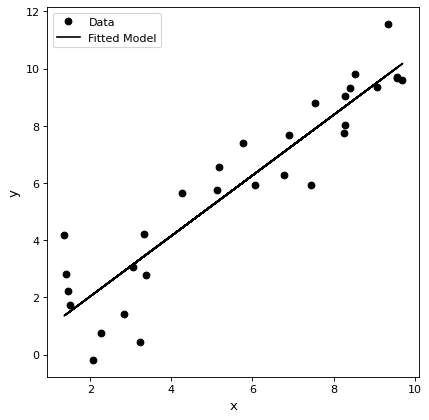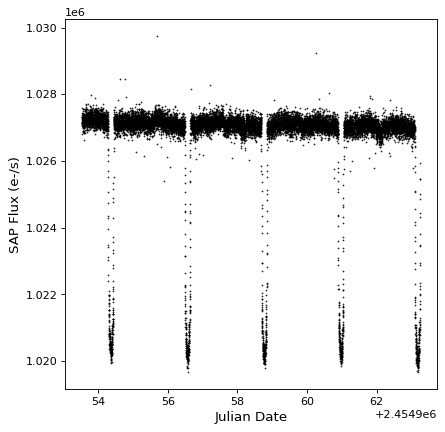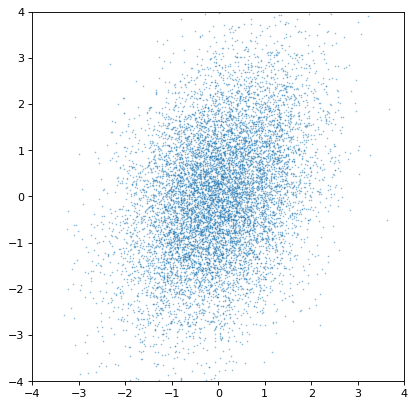# N-dimensional datasets (astropy.nddata)¶

## Introduction¶

The nddata package provides classes to represent images and other gridded data, some essential functions for manipulating images, and the infrastructure for package developers who wish to include support for the image classes.

## Getting started¶

### NDData¶

The primary purpose of NDData is to act as a container for data, metadata, and other related information like a mask.

An NDData object can be instantiated by passing it an n-dimensional numpy array:

>>> import numpy as np
>>> from astropy.nddata import NDData
>>> array = np.zeros((12, 12, 12))  # a 3-dimensional array with all zeros
>>> ndd1 = NDData(array)


or something that can be converted to an numpy.ndarray:

>>> ndd2 = NDData([1, 2, 3, 4])
>>> ndd2
NDData([1, 2, 3, 4])


and can be accessed again via the data attribute:

>>> ndd2.data
array([1, 2, 3, 4])


It also supports additional properties like a unit or mask for the data, a wcs (world coordinate system) and uncertainty of the data and additional meta attributes:

>>> data = np.array([1,2,3,4])
>>> mask = data > 2
>>> unit = 'erg / s'
>>> from astropy.nddata import StdDevUncertainty
>>> uncertainty = StdDevUncertainty(np.sqrt(data)) # representing standard deviation
>>> meta = {'object': 'fictional data.'}
>>> from astropy.coordinates import SkyCoord
>>> wcs = SkyCoord('00h42m44.3s', '+41d16m09s')
>>> ndd = NDData(data, mask=mask, unit=unit, uncertainty=uncertainty,
...              meta=meta, wcs=wcs)
>>> ndd
NDData([1, 2, 3, 4])


The representation only displays the data; the other attributes need to be accessed directly, for example ndd.mask to access the mask.

### NDDataRef¶

Building upon this pure container NDDataRef implements:

• a read and write method to access astropy’s unified file io interface.
• simple arithmetics like addition, subtraction, division and multiplication.
• slicing.

Instances are created in the same way:

>>> from astropy.nddata import NDDataRef
>>> ndd = NDDataRef(ndd)
>>> ndd
NDDataRef([1, 2, 3, 4])


But also support arithmetic (NDData Arithmetic) like addition:

>>> import astropy.units as u
>>> ndd2 = ndd.add([4, -3.5, 3, 2.5] * u.erg / u.s)
>>> ndd2
NDDataRef([ 5. , -1.5,  6. ,  6.5])


Because these operations have a wide range of options these are not available using arithmetic operators like +.

Slicing or indexing (Slicing and Indexing NDData) is possible (issuing warnings if some attribute cannot be sliced):

>>> ndd2[2:]  # discard the first two elements
INFO: wcs cannot be sliced. [astropy.nddata.mixins.ndslicing]
NDDataRef([6. , 6.5])
>>> ndd2   # get the second element
INFO: wcs cannot be sliced. [astropy.nddata.mixins.ndslicing]
NDDataRef(-1.5)


### Working with two-dimensional data like images¶

Though the nddata package supports any kind of gridded data, this introduction will focus on the use of nddata for two-dimensional images. To get started, we’ll construct a two-dimensional image with a few sources, some Gaussian noise, and a “cosmic ray” which we will later mask out:

>>> import numpy as np
>>> from astropy.modeling.models import Gaussian2D
>>> y, x = np.mgrid[0:500, 0:600]
>>> data = (Gaussian2D(1, 150, 100, 20, 10, theta=0.5)(x, y) +
...         Gaussian2D(0.5, 400, 300, 8, 12, theta=1.2)(x,y) +
...         Gaussian2D(0.75, 250, 400, 5, 7, theta=0.23)(x,y) +
...         Gaussian2D(0.9, 525, 150, 3, 3)(x,y) +
...         Gaussian2D(0.6, 200, 225, 3, 3)(x,y))
>>> data += 0.01 * np.random.randn(500, 600)
>>> cosmic_ray_value = 0.997
>>> data[100, 300:310] = cosmic_ray_value


This image has a large “galaxy” in the lower left and the “cosmic ray” is the horizontal line in the lower middle of the image:

>>> import matplotlib.pyplot as plt
>>> plt.imshow(data, origin='lower')


(png, svg, pdf)The “cosmic ray” can be masked out, in this simple test image, like this:

>>> mask = (data == cosmic_ray_value)


### CCDData class for images¶

The CCDData object, like the other objects in this package, can store the data, a mask, and metadata. The CCDData object requires that a unit be specified:

>>> from astropy.nddata import CCDData
...               meta={'object': 'fake galaxy', 'filter': 'R'},


### Slicing¶

Slicing the works the way you would expect, with the mask and, if present, WCS, sliced appropriately also:

>>> ccd2 = ccd[:200, :]
>>> ccd2.data.shape
(200, 600)
(200, 600)
>>> # Show the mask in a region around the cosmic ray:
array([[False, False, False, False, False, False, False, False, False,
False, False, False],
[False,  True,  True,  True,  True,  True,  True,  True,  True,
True,  True, False],
[False, False, False, False, False, False, False, False, False,
False, False, False]]...)


For many applications it may be more convenient to use Cutout2D, described in image_utilities.

### Image arithmetic, including uncertainty¶

Methods are provided for basic arithmetic operations between images, including propagation of uncertainties. Three uncertainty types are supported: variance (VarianceUncertainty), standard deviation (StdDevUncertainty) and inverse variance (InverseVariance). The example below creates an uncertainty that is simply Poisson error, stored as a variance:

>>> from astropy.nddata import VarianceUncertainty
>>> poisson_noise = np.ma.sqrt(np.ma.abs(ccd.data))
>>> ccd.uncertainty = VarianceUncertainty(poisson_noise ** 2)


As a convenience, the uncertainty can also be set with a numpy array. In that case, the uncertainty is assumed to be the standard deviation:

>>> ccd.uncertainty = poisson_noise
INFO: array provided for uncertainty; assuming it is a StdDevUncertainty. [astropy.nddata.ccddata]


If we make a copy of the image and add that to the original, the uncertainty changes as expected:

>>> ccd2 = ccd.copy()
>>> added_ccds.uncertainty.array[0, 0] / ccd.uncertainty.array[0, 0] / np.sqrt(2)
0.99999999999999989


### Reading and writing¶

A CCDData can be saved to a FITS file:

>>> ccd.write('test_file.fits')


and can also be read in from a FITS file:

>>> ccd2 = CCDData.read('test_file.fits')


Note the unit is stored in the BUNIT keyword in the header on saving, and is read from the header if it is present.

### Image utilities¶

#### Cutouts¶

Though slicing directly is one way to extract a subframe, Cutout2D provides more convenient access to cutouts from the data. The example below pulls out the large “galaxy” in the lower left of the image, with the center of the cutout at position:

>>> from astropy.nddata import Cutout2D
>>> position = (149.7, 100.1)
>>> size = (81, 101)     # pixels
>>> cutout = Cutout2D(ccd, position, size)
>>> plt.imshow(cutout.data, origin='lower')


(png, svg, pdf)This cutout can also plot itself on the original image:

>>> plt.imshow(ccd, origin='lower')
>>> cutout.plot_on_original(color='white')


(png, svg, pdf)The cutout also provides methods for find pixel coordinates in the original or in the cutout; recall that position is the center of the cutout in the original image:

>>> position
(149.7, 100.1)
>>> cutout.to_cutout_position(position)
(49.7, 40.099999999999994)
>>> cutout.to_original_position((49.7, 40.099999999999994))
(149.7, 100.1)


For more details, including constructing a cutout from world coordinates and the options for handling cutouts that go beyond the bounds of the original image, see 2D Cutout Images.

#### Image resizing¶

The functions block_reduce and block_replicate resize images. The example below reduces the size of the image by a factor of 4. Note that the result is a numpy.ndarray; the mask, metadata, etc are discarded:

>>> from astropy.nddata import block_reduce, block_replicate
>>> smaller = block_reduce(ccd, 4)
>>> smaller
array(...)
>>> plt.imshow(smaller, origin='lower')


(png, svg, pdf)By default, both block_reduce and block_replicate conserve flux.

### Other image classes¶

There are two less restrictive classes, NDDataArray and NDDataRef, that can be used to hold image data. They are primarily of interest to those who may want to create their own image class by subclassing from one of the classes in the nddata package. The main differences between them are:

• NDDataRef can be sliced and has methods for basic arithmetic operations, but the user needs to use one of the uncertainty classes to define an uncertainty. See NDDataRef for more detail. Most of its properties must be set when the object is created because they are not mutable.
• NDDataArray extends NDDataRef by adding the methods necessary to all it to behave like a numpy array in expressions and adds setters for several properties. It lacks the ability to automatically recognize and read data from FITS files and does not attempt to automatically set the WCS property.
• CCDData extends NDDataArray by setting up a default uncertainty class, sets up straightforward read/write to FITS files, automatically sets up a WCS property.

### More general gridded data class¶

There are two additional classes in the nddata package that are of interest primarily to people that either need a custom image class that goes beyond the classes discussed so far or who are working with gridded data that is not an image.

• NDData is a container class for holding general gridded data. It includes a handful of basic attributes, but no slicing or arithmetic. More information about this class is in NDData.
• NDDataBase is an abstract base class that developers of new gridded data classes can subclass to declare that the new class follows the NDData interface. More details are in Subclassing.

The list of packages below that use the nddata framework is intended to be useful to either people writing their own image classes or for those looking for an image class that goes beyond what CCDData does.

## Performance Tips¶

• Using the uncertainty class VarianceUncertainty will be somewhat more efficient than the other two uncertainty classes, InverseVariance and StdDevUncertainty. The latter two are converted to variance for the purposes of error propagation and then converted from variance back to the original uncertainty type. The performance difference should be small.
• When possible, mask values by setting them to np.nan and use the Numpy functions and methods that automatically exclude np.nan, like np.nanmedian and np.nanstd. That will typically be much faster than using numpy.ma.MaskedArray.

## Reference/API¶

### astropy.nddata Package¶

The astropy.nddata subpackage provides the NDData class and related tools to manage n-dimensional array-based data (e.g. CCD images, IFU Data, grid-based simulation data, …). This is more than just numpy.ndarray objects, because it provides metadata that cannot be easily provided by a single array.

#### Functions¶

 add_array(array_large, array_small, position) Add a smaller array at a given position in a larger array. bitfield_to_boolean_mask(bitfield[, …]) Converts an array of bit fields to a boolean (or integer) mask array according to a bit mask constructed from the supplied bit flags (see ignore_flags parameter). block_reduce(data, block_size[, func]) Downsample a data array by applying a function to local blocks. block_replicate(data, block_size[, conserve_sum]) Upsample a data array by block replication. extract_array(array_large, shape, position) Extract a smaller array of the given shape and position from a larger array. fits_ccddata_reader(filename[, hdu, unit, …]) Generate a CCDData object from a FITS file. fits_ccddata_writer(ccd_data, filename[, …]) Write CCDData object to FITS file. interpret_bit_flags(bit_flags[, flip_bits]) Converts input bit flags to a single integer value (bit mask) or None. overlap_slices(large_array_shape, …[, mode]) Get slices for the overlapping part of a small and a large array. subpixel_indices(position, subsampling) Convert decimal points to indices, given a subsampling factor. support_nddata([_func, accepts, repack, …]) Decorator to wrap functions that could accept an NDData instance with its properties passed as function arguments.

#### Classes¶

 CCDData(*args, **kwd) A class describing basic CCD data. Conf Configuration parameters for astropy.nddata. Cutout2D(data, position, size[, wcs, mode, …]) Create a cutout object from a 2D array. FlagCollection(*args, **kwargs) The purpose of this class is to provide a dictionary for containing arrays of flags for the NDData class. IncompatibleUncertaintiesException This exception should be used to indicate cases in which uncertainties with two different classes can not be propagated. InverseVariance([array, copy, unit]) Inverse variance uncertainty assuming first order Gaussian error propagation. MissingDataAssociationException This exception should be used to indicate that an uncertainty instance has not been associated with a parent NDData object. NDArithmeticMixin Mixin class to add arithmetic to an NDData object. NDData(data[, uncertainty, mask, wcs, meta, …]) A container for numpy.ndarray-based datasets, using the NDDataBase interface. NDDataArray(data, *args[, flags]) An NDData object with arithmetic. NDDataBase() Base metaclass that defines the interface for N-dimensional datasets with associated meta information used in astropy. NDDataRef(data[, uncertainty, mask, wcs, …]) Implements NDData with all Mixins. NDIOMixin Mixin class to connect NDData to the astropy input/output registry. NDSlicingMixin Mixin to provide slicing on objects using the NDData interface. NDUncertainty([array, copy, unit]) This is the metaclass for uncertainty classes used with NDData. NoOverlapError Raised when determining the overlap of non-overlapping arrays. PartialOverlapError Raised when arrays only partially overlap. StdDevUncertainty([array, copy, unit]) Standard deviation uncertainty assuming first order gaussian error propagation. UnknownUncertainty([array, copy, unit]) This class implements any unknown uncertainty type. VarianceUncertainty([array, copy, unit]) Variance uncertainty assuming first order Gaussian error propagation.

A module that provides functions for manipulating bit masks and data quality (DQ) arrays.

#### Functions¶

 bitfield_to_boolean_mask(bitfield[, …]) Converts an array of bit fields to a boolean (or integer) mask array according to a bit mask constructed from the supplied bit flags (see ignore_flags parameter). interpret_bit_flags(bit_flags[, flip_bits]) Converts input bit flags to a single integer value (bit mask) or None.

### astropy.nddata.utils Module¶

This module includes helper functions for array operations.

#### Functions¶

 extract_array(array_large, shape, position) Extract a smaller array of the given shape and position from a larger array. add_array(array_large, array_small, position) Add a smaller array at a given position in a larger array. subpixel_indices(position, subsampling) Convert decimal points to indices, given a subsampling factor. overlap_slices(large_array_shape, …[, mode]) Get slices for the overlapping part of a small and a large array. block_reduce(data, block_size[, func]) Downsample a data array by applying a function to local blocks. block_replicate(data, block_size[, conserve_sum]) Upsample a data array by block replication.

#### Classes¶

 NoOverlapError Raised when determining the overlap of non-overlapping arrays. PartialOverlapError Raised when arrays only partially overlap. Cutout2D(data, position, size[, wcs, mode, …]) Create a cutout object from a 2D array.